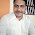Type Here to Get Search Results !

# CBSE UGC NET July 2016 Answer Key for LIS Paper 3 Fully Modified

3

This is a Tentative Answer Key for UGC NET July 2016 Paper 3 Library and Information Science
If you have a doubt regarding answers please give a comment or mail me to thiru.nethra@gmail.com

 1. Second Law 2. Electronic Resources 3. IFLA 4. Dr. Abdul Kalam 5. James I Wyer 6. Arithmetic progression 7. Section 8 8. Library of Congress , with support of UNESCO 9. Five laws of library science 10. Online database of freely available journal information 11. The first issue of periodical 12. WCB Sayers 13. Main Series Entry 14. Edgar F. Codd 15. LibriVox 16. the organisation is competitive and enabled to meet future needs as they occur 17. Scalar Chain 18. Facebook 19. Agriculture 20. Stanford University 21. a, b and d are correct 22. a, b and c are correct 23. a and b are correct 24. a and b are correct 25. a, b, d are correct 26. a, b, c are correct 27. a and b are correct 28. b, c, d are correct 29. b, c, d are correct 30. a, b and c are correct* 31. a, c, d are correct 32. a, b and d are correct 33. c & d are correct 34. b and c are correct 35. b, d, c and a 36. c, d, a and b (given answers are wrong) 37. b, d, c, a 38. d, b, a, c 39. b, a, d, c 40. d, c, a, b 41. c, b, a, d* 42. c, a, d, b 43. b, d, a, c 44. ii, iii, iv, i 45. * 46. iii, iv, ii, i 47. iv, i, iii, ii 48. ii, i, iii, iv 49. iv, iii, i, ii 50. iii, i, ii, iv 51. iv, iii, ii, i 52. ii, iii, i, iv 53. ii, iii, iv, i 54. ii, i, iv, iii 55. iii, i, iv, ii 56. iv, i, iii, ii* 57. iv, iii, i, ii 58. ii, iii, iv, i 59. iii, ii, iv, i 60. Both (A) and (R) are true 61. (A) is true, but (R) is false 62. Both (A) and (R) are true 63. (A) is true, but (R) is false 64. Both (A) and (R) are true 65. Both (A) and (R) are true 66. (A) is true, but (R) is false 67. Both (A) and (R) are true 68. Both (A) and (R) are true 69. Both (A) and (R) are true 70. Both (A) and (R) are true 71. Both (A) and (R) are true 72. (A) is true, but (R) is false 73. Both (A) and (R) are true 74. (A) is false, but (R) is true 75. Both (A) and (R) are true
Tags

1.6th Question Exponential growuth refrers to geometric progression??

2.Thanks a lot sir

3.Hello Sir,